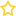## Mathematics

Sort by:

This Course is FREE

### Course Overview

This Grade 2 Math course emphasizes base-ten notation, fluency with addition and subtraction, using standard units of measure, and describing and analyzing shapes.

### Sample Lesson - Subtraction Review(0 Votes)

#### This Course is FREE

38666 Viewers
This Course is FREE

### Course Overview

The Acellus Grade 5 Math course is designed to give students additional experience with basic mathematical operations (addition, subtraction, multiplication, and division). Besides working with larger whole numbers, in this course students focus on...(0 Votes)

#### This Course is FREE

45170 Viewers
This Course is FREE

### Course Overview

The Acellus Grade 1 Math course focuses on addition, subtraction through the number 20, whole number relationships and place value, linear measurement, measuring lengths, and geometric shapes.

### Course Objectives & Student Learning Outcomes

Upon completion of...(0 Votes)

#### This Course is FREE

40799 Viewers
This Course is FREE

### Course Overview

This Grade 4 Math course emphasizes multi-digit multiplication and division, fractions, and analysis and classification of geometric figures.

### Course Objectives & Student Learning Outcomes

Upon completion of Acellus Grade 4 Math, students will be able to:...(0 Votes)

#### This Course is FREE

40324 Viewers
This Course is FREE

### Course Overview

This Grade 3 Math course focuses on multiplication and division up to the number 100, fractions, the structure of rectangular arrays and area, and two-dimensional shapes.

### Sample Lesson - Round to the Nearest Ten(0 Votes)

#### This Course is FREE

42889 Viewers
##### Kindergarten Math
This Course is FREE

### Course Overview

Grade K Math leads students through the rudimentary concepts required for math, preparing them for mathematical operations. The use of colorful and varied manipulatives and graphics in the video lessons adds variety and helps students...

### Kindergarten Math(0 Votes)

47423 Viewers